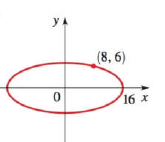Chapter 12.2, Problem 33E### Algebra and Trigonometry (MindTap ...

4th Edition
James Stewart + 2 others
ISBN: 9781305071742

#### Solutions

Chapter
Section### Algebra and Trigonometry (MindTap ...

4th Edition
James Stewart + 2 others
ISBN: 9781305071742
Textbook Problem

# 29-34 Finding the Equation of an Ellipse Find an equation for the ellipse whose graph is shown.To determine

The equation for the ellipse given in graph.

Explanation

Given:

The given graph of an ellipse is,

Figure (1)

From the graph,

The value of a is,

a=16.

The x and y coordinate which lie on ellipse are,

(x,y)=(8,6).

Approach:

The basic equation for an ellipse with a horizontal major axis which is used is as follows,

x2a2+y2b2=1

Where a and b are constant values.

Calculation:

Consider the standard equation of an ellipse,

x2a2+y2b2=1 …… (1)

Substitute 16 for a, 8 for x and 6 for y in the above equation to calculate the value of b.

(8)2(16)2+(6)2b2=164256+36b2=136b2=16425636b2=114

Further solve the above equation

### Still sussing out bartleby?

Check out a sample textbook solution.

See a sample solution

#### The Solution to Your Study Problems

Bartleby provides explanations to thousands of textbook problems written by our experts, many with advanced degrees!

Get Started